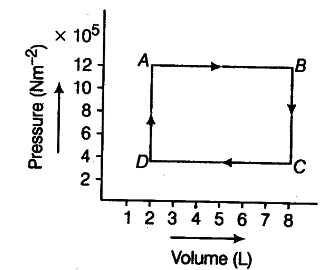# The figure given below represents p-V diagram

The figure given below represents p-V diagram of different stages of a thermodynamic process. Calculate the work done in each stage and also the net work done in the complete cyclic process.